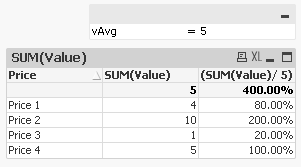# QlikView App Development

Discussion Board for collaboration related to QlikView App Development.

Contributor

## How to calculate a relative %

Dear Gurus,

Is it any way to calculate the relative % of the total for each line in a straight table?

For example:

Prices            % Participation (the one I want to calculate)

Average Price     5                           5

Price 1                4                           80%

Price 2               10                          200%

Price 3                1                           20%

Price 4                5                           100%

Thanks!!

Regards,

Jordi

1 Solution

Accepted SolutionsMVP

## Re: How to calculate a relative %

Check !

You can also use expression as

SUM(Value)/Avg({1}total Value)

7 Replies
Esteemed Contributor III

## Re: How to calculate a relative %

u can give ur exp

sum( prices)

and go to number tab and chk  show in %

Esteemed Contributor III

## Re: How to calculate a relative %

or else in expression tab   u will find relative option u can chk thereEmployee

## Re: How to calculate a relative %

sum(prices) / sum(total prices)MVP

## Re: How to calculate a relative %

Check !

You can also use expression as

SUM(Value)/Avg({1}total Value)MVP

## Re: How to calculate a relative %

What expression are you using.

Regards

AnandMVP

## Re: How to calculate a relative %

You are using variable also

Ex:-

Let vAvg = 5

Dim:- Price

Expre:- (SUM(Value)/ \$(vAvg))Regards

Anand

Contributor

## Re: How to calculate a relative %

Thank you! It solved my question!

Regards,

Jordi